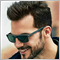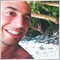# MT5 Issue : cannot get 1Day ATR at different Timeframe.164

Hi guys,

I need to get ATR value of Daily period at any timeframe but I get 4806 error code. I don't know how to tackle it.

At 1Day Period I can get ATR values, but I need to get 1Day ATR values at any timeframe.

when I change Timeframe, I get BarsCalculated() returned -1, error code 4806.

```//+------------------------------------------------------------------+
//|                                                    Demo_iATR.mq5 |
//|                        Copyright 2011, MetaQuotes Software Corp. |
//|                                              https://www.mql5.com |
//+------------------------------------------------------------------+
#property version   "1.00"
#property indicator_chart_window
#property indicator_plots   0

//--- input parameters
input int                  atr_period=14;          // period of calculation

//--- indicator buffer
double         iATRBuffer[];

int    handle;
int    bars_calculated=0;

//+------------------------------------------------------------------+
//| Custom indicator initialization function                         |
//+------------------------------------------------------------------+
int OnInit()
{
ArrayInitialize(iATRBuffer,EMPTY_VALUE);
ArraySetAsSeries(iATRBuffer,true);

//--- create handle of the indicator
handle=iATR(_Symbol, PERIOD_D1, atr_period);

//--- if the handle is not created
if(handle==INVALID_HANDLE)
{
//--- tell about the failure and output the error code
Print("Failed to create handle of the iATR indicator , error code ", GetLastError());
//--- the indicator is stopped early
return(INIT_FAILED);
}

//--- normal initialization of the indicator
return(INIT_SUCCEEDED);
}
//+------------------------------------------------------------------+
//| Custom indicator iteration function                              |
//+------------------------------------------------------------------+
int OnCalculate(const int rates_total,
const int prev_calculated,
const datetime &time[],
const double &open[],
const double &high[],
const double &low[],
const double &close[],
const long &tick_volume[],
const long &volume[],
{
//--- number of values copied from the iATR indicator
int values_to_copy;

//--- determine the number of values calculated in the indicator
int calculated=BarsCalculated(handle);
if(calculated<=0)
{
PrintFormat("BarsCalculated() returned %d, error code %d",calculated,GetLastError());
return(0);
}

//--- if it is the first start of calculation of the indicator or if the number of values in the iATR indicator changed
//---or if it is necessary to calculated the indicator for two or more bars (it means something has changed in the price history)
if(prev_calculated==0 || calculated!=bars_calculated || rates_total>prev_calculated+1)
{
//--- if the iATRBuffer array is greater than the number of values in the iATR indicator for symbol/period, then we don't copy everything
//--- otherwise, we copy less than the size of indicator buffers
if(calculated>rates_total) values_to_copy=rates_total;
else                       values_to_copy=calculated;
}
else
{
//--- it means that it's not the first time of the indicator calculation, and since the last call of OnCalculate()
//--- for calculation not more than one bar is added
values_to_copy=(rates_total-prev_calculated)+1;
}

//--- fill the iATRBuffer array with values of the Average True Range indicator
//--- if FillArrayFromBuffer returns false, it means the information is nor ready yet, quit operation
if(!FillArrayFromBuffer(iATRBuffer,handle,values_to_copy)) return(0);

else
{
//---- print ATR values
for(int i=0; i<ArraySize(iATRBuffer); i++)
Print(i," -> ",iATRBuffer[i]);
}

//--- memorize the number of values in the Average True Range indicator
bars_calculated=calculated;

//--- return the prev_calculated value for the next call
return(rates_total);
}
//+------------------------------------------------------------------+
//| Filling indicator buffers from the iATR indicator                |
//+------------------------------------------------------------------+
bool FillArrayFromBuffer(double &values[],  // indicator buffer for ATR values
int ind_handle,    // handle of the iATR indicator
int amount         // number of copied values
)
{
//--- reset error code
ResetLastError();
//--- fill a part of the iATRBuffer array with values from the indicator buffer that has 0 index
if(CopyBuffer(ind_handle,0,0,amount,values)<0)
{
//--- if the copying fails, tell the error code
PrintFormat("Failed to copy data from the iATR indicator, error code %d",GetLastError());
//--- quit with zero result - it means that the indicator is considered as not calculated
return(false);
}
//--- everything is fine
return(true);
}
//+------------------------------------------------------------------+
//| Indicator deinitialization function                              |
//+------------------------------------------------------------------+
void OnDeinit(const int reason)
{
if(handle!=INVALID_HANDLE)
IndicatorRelease(handle);
//--- clear the chart after deleting the indicator
Comment("");
}```164

Do you have any tips to solve the problem?5032

From what I've seen it can occur that on first OnCalculate call there can be some issues in getting other timeframes/symbols datas but on next calls it works well.
You are also handling the error returning 0, so I guess that your indicator works well.
Am I wrong?

From documentation: https://www.mql5.com/en/docs/series/timeseries_access#synchronized

For Expert Advisors and indicators, it is better to use the event model of handling. If during handling of OnTick() or OnCalculate() event, data receipt for the required timeseries failed, you should exit the event handler, relying on the access availability during the next call of the handler.164

Fabio Cavalloni:
From what I've seen it can occur that on first OnCalculate call there can be some issues in getting other timeframes/symbols datas but on next calls it works well.
You are also handling the error returning 0, so I guess that your indicator works well.
Am I wrong?

From documentation: https://www.mql5.com/en/docs/series/timeseries_access#synchronized

For Expert Advisors and indicators, it is better to use the event model of handling. If during handling of OnTick() or OnCalculate() event, data receipt for the required timeseries failed, you should exit the event handler, relying on the access availability during the next call of the handler.

Actually I also use the indicator when Market is Closed. At this time OnCalculate() called only once.241

Try this indicator, it give same result as ATR and is MTF

https://www.mql5.com/en/code/20317164

markosb1979:

Try this indicator, it give same result as ATR and is MTF

https://www.mql5.com/en/code/20317

thanks bro